Z Score Table

What is Z Score Table? - Z Score Formula and Negative Z Score Table

Z-score can be defined as the number of standard deviations from the mean. A data point is a measure of how many standard deviations below or above mean. A raw score as a Z-score can also be called as a standard score and it can be placed on a normal distribution curve. Z-scores range from -3 standard deviations up to +3 standards.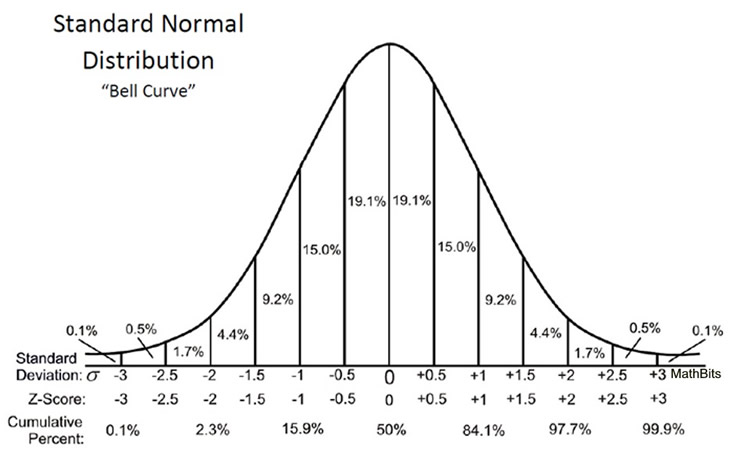A Z-score can help us in determining the difference or the distance between a value and the mean value. When you "standardize" a variable, its mean becomes zero and its standard deviation becomes one.

How to calculate Z-score?

For a sample, the basic z- score formula is
z = (x – μ) / σ
Where,
μ is the mean value
x is the test value
σ is the standard deviation

Or another formula can be used,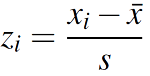Where,

x̄ is the sample mean
s is the sample standard deviation.

Example
Let's take an example and understand this better. Below is an example problem.
You have a test score of 190. The test has a mean (μ) of 140 and a standard deviation (σ) of 30. Assuming it is a normal distribution, your z score would be.

Solution
From the question above we can deduce that,
The value of x is 190 (test score)
The value of mean (μ) is 140
And the value of standard deviation (σ) is 30
Putting the values in the equation mentioned above,
z = (x – μ) / σ
=( 190 – 140) / 30 = 1.6
Z Score Formula: Standard Error of the Mean

When you have multiple samples and want to describe the standard deviation of those sample means (the standard error), use this z score formula:

z = (x – μ) / (σ / √n)
This z-score will tell you how many standard errors are there between the sample mean and the population means.

Z-score tables: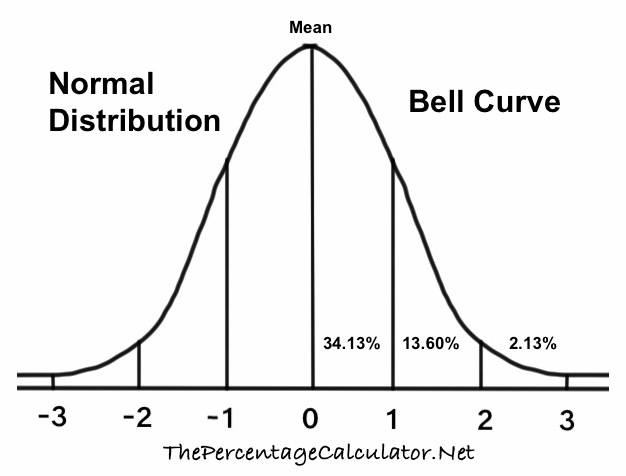Area under a normal curve:

To find a specific area under a normal curve, first, find the z-score of the data value and then use a Z-Score Table to find the area. A Z-Score Table is a table which shows the percentage of values (or area percentage) to the left of a given z-score on a standard normal distribution.

There are two Z-score tables:

• 1) Positive Z- score table

• A positive Z-score means that the observed value is above the mean of total values.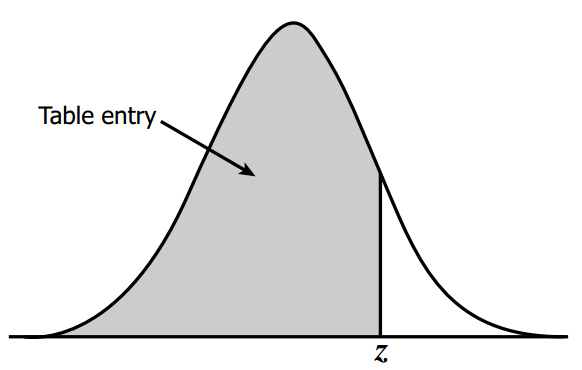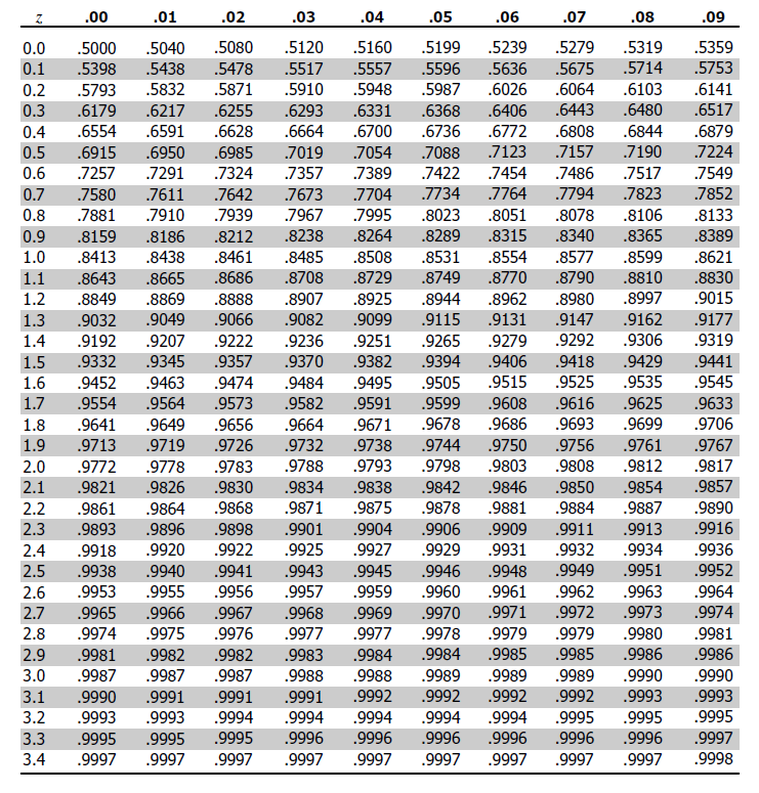• 2) Negative Z-scores table

• A negative Z-score value indicates the observed value is below the mean of total values.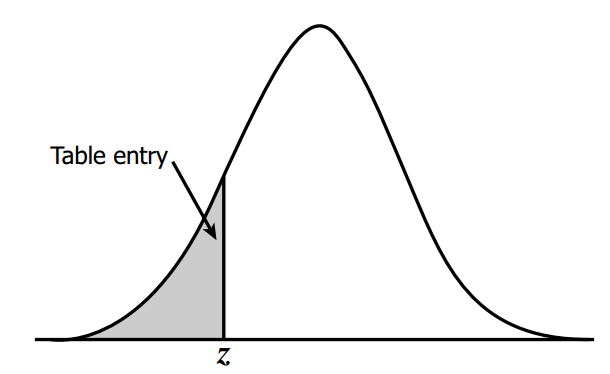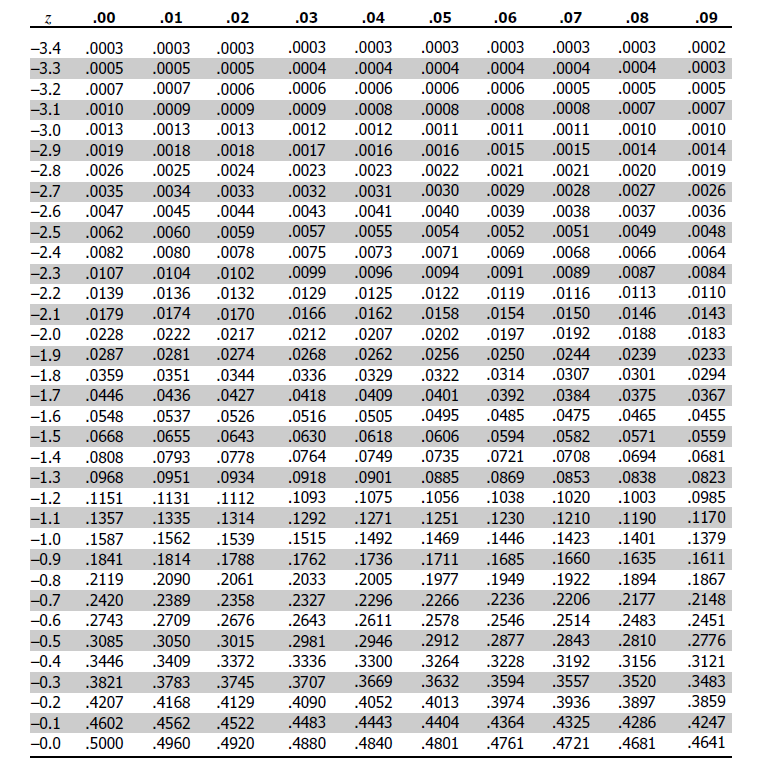These tables are specifically designed for a standard normal distribution, which has a mean of 0 and a standard deviation of 1.

The table given above is designed specifically for standard normal distribution. The mean of these tables is 0 and 1 is their standard deviation.

• • In the first column of the table, we can find out the number of standard deviations either above or below the mean value to one decimal place. (The integer part and the first decimal of Z-score is present in the row label)

• • Across the topmost row of the table, the part which denotes the z-score denotes the hundredth.

• • Then, the intersecting point of the columns and rows provides us with the area under the normal curve or the probability.

• Let’s consider the following example

Imagine a group of 300 applicants who took a math test. Sarah got 700 points (X) out of 1000. The average score was 500 (µ) and the standard deviation was 120 (σ). Find out how well Sarah performed compared to her peers.

SOLUTION

• 1) Find out Z-score

• From the above-given data, we can deduce that
The value of x is 700
The value of μ is 600
And the value of σ is 150
Z score = (x – μ) / σ
= (700-600) / 150
= 0.68

• 2) Using the Z-score table we can find out how well she performed relative to her peers. Now we need to determine the percentage of peers whose score goes higher and lower then that of the scores of Sarah.

• In this example the Z-score calculated is positive, therefore we refer to all the positive values in the Z-score table.
There are certain steps to be followed while using the Z score table.

The steps to be followed while referring to the Z-scale table are,

• 1) First, Find the first two digits on the y-axis (in our example the first two digits are 0.6).

• 2) Then, go to the x-axis in order to find the second decimal number (according to our example it is 0.7) the number is 0.7486.

• 3) Next, multiply this number by 100 in order to get the percentage

• So,
0.7486 x 100 = 74.86%.

• 4) This means that nearly 75% of the students scored lower than Sarah and only 25% scored higher than her. 75% out of 200 students is 150. This means that Sarah did better than 150 students.

• So, this is how to solve a question based on Z-score tables.

Z-score formats

The z-score tables come in different formats. Below are the two most popular z-score formats,

• 1. Yielding area or the probability

• 2. The first format helps us in deducing the area or the probability. Start at the mean and then go towards the right of the mean till the required z-score. These tables are generally called as “cumulative from the mean”. The table works by using half of the area under the normal curve and the user has made sure that they take this into consideration and make suitable adjustments while using the table. Only positive z-scores are present in this table. Cumulative from left

• This table format helps in deducing area or the probability ranging from the negative infinity (the last left) and then going right above the required z-score. These tables thus are called as “cumulative from the left”. The table works with the whole area under the normal curve and does not require much adjustment in comparison to the first option. Both positive z-score and negative z-score values can be used under this format.

Z scores and Standard Deviations

A z-score is basically the number of standard deviations from the mean value of the reference population (a population whose known values have been recorded, like in these charts the CDC compiles about people’s weights). For example:

A z-score of 1 is 1 and the standard deviation is present above the mean.
A score of -2 is -2 and the standard deviation is present below the mean.
A score of 1.8 is 1.8 and the standard deviation is present above the mean.
A z-score tells you where exactly the score lies on a normal distribution curve. A z-score of zero tells you the values are exactly average while a score of +3 tells you that the value is much higher than average.

Applications of z-scores

In a normal distribution of a variable, the center of distribution is the mean and the standard deviation is an indication of the existing variability.

One can use the z-table to find the areas for a calculated z-score if the one is interested in determining the probability of a specific value in order to determine the area under any normal distribution. This can help one know the chances of a value to occur. It is also to be noted that not all z-score tables are the same.